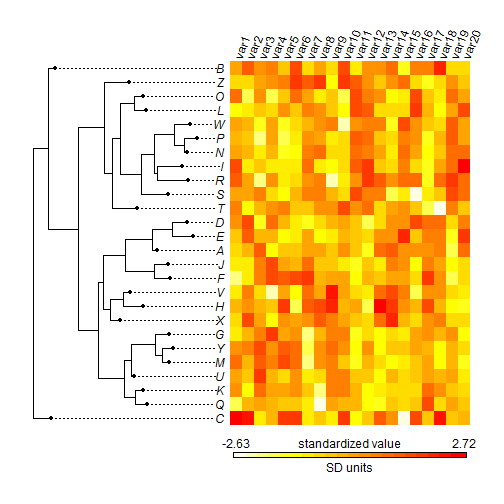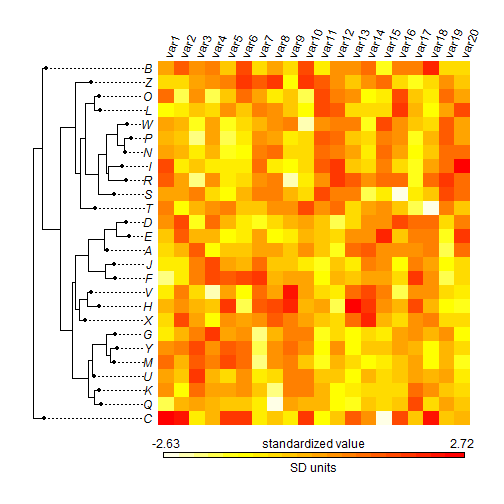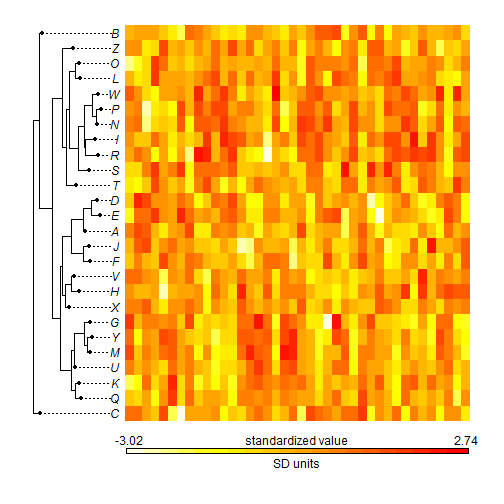## Wednesday, February 3, 2016

### More options & user control for `phylo.heatmap`

In response to a user comment I have just added some user control features to `phylo.heatmap` (a function that I have described previously in some recent posts: 1, 2, 3).

The main user control feature is the ability to control the fraction of horizontal space dedicated to the tree and heatmap, respectively. This is controlled by an optional argument, `split`, which is just a numeric vector with two elements that is most sensibly considered to be the fraction of space for each subplot (although it can also be the ratio of space, e.g., `c(1,2)` would give 2 × as much space to the heatmap as to the tree).

Here is a demo:

``````library(phytools)
``````
``````## Loading required package: ape
##
##  # ATTENTION: maps v3.0 has an updated 'world' map.        #
##  # Many country borders and names have changed since 1990. #
##  # Type '?world' or 'news(package="maps")'. See README_v3. #
``````
``````tree<-rtree(n=26,tip.label=LETTERS)
X<-fastBM(tree,nsim=20)
phylo.heatmap(tree,X,standardize=TRUE)
````````````phylo.heatmap(tree,X,split=c(1,2),standardize=TRUE)
``````I also fixed a bug that meant the two optional logical arguments `labels` (to turn on or off the trait column labels) and `legend` (to turn on or off the legend) were not working properly. In fact, the arguments only resized the plot area, but did not actually turn off either element. Here is an example without the trait labels:

``````X<-fastBM(tree,nsim=40)
phylo.heatmap(tree,X,labels=FALSE,split=c(0.25,0.75),standardize=TRUE)
``````That's it.

1.2.